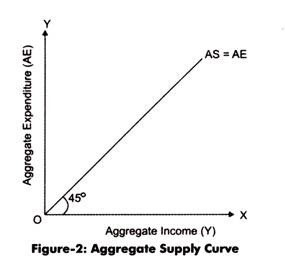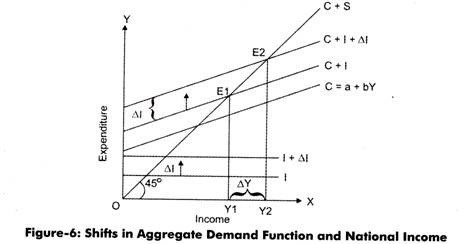# Simple Keynesian Model of Income Determination

According to Keynes, there can be different sources of national income, such as government, foreign trade, individuals, businesses and trusts.

For determining national income, Keynes had divided the different sources of income into four sectors namely’ household sector, business sector, government sector, and foreign sector.

He prepared three models for the determination of national income, which are shown in Figure-1:The two-sector model of economy involves households and businesses only, while three-sector model represents households businesses, and government. On the other hand, the four-sector model contains households, businesses, government, and foreign sector.Let us discuss these three types of models of income determination given by Keynes.

Determination of National Income in Two-Sector Economy:

The determination of level of national income in the two-sector economy is based on an assumption that two-sector economy is an economy where there is no intervention of the government and foreign trade.

Apart from this, an economy can be a two-sector economy if it satisfies the following assumptions:

1. Comprises only two sectors, namely, households and businesses. The households are the owners of factors of production and provide factor services to businesses to earn their livelihood in the form of wages, rents, interest, and profits. In addition the households are the consumers of final goods and services produced by businesses. On the other hand, businesses purchase factor services from households to produce goods and services and sell it to households.
2. Does not have government interference. If government is there, it does not have any role to play in the economic activity of a country. For example, in the two-sector economy, the government is not involved in activities, such as taxation, expenditure, and consumption.
3. Comprises a closed economy in which the foreign trade does not exist. In other words, import and export services are absent in such an economy.
4. Contains no profit that is undistributed or savings by the organization. In other words, the profit earned by an organization is completely distributed in the form of dividends among shareholders.
5. Keeps the prices of goods and services, supply of factors of production, and production technique constant throughout the life cycle of organization.

Keynes believed that there are two major factors that determine the national income of a country. These two factors are Aggregate Supply (AS) and Aggregate Demand (AD) of goods and services.

In addition, he believed that the equilibrium level of national income can be estimated when AD=AS. Before representing the relationship between AS and AD on a graph, let us understand these two concepts in detail.

### Aggregate Supply:

AS can be defined as total value of goods and services produced and supplied at a particular point of time. It comprises consumer goods as well as producer goods. When goods and services produced at a particular point of time is multiplied by the respective prices of goods and services, it provides the total value of the national output. The national output is the aggregate supply in the form of money value. The Keynesian AS curve is drawn based on an assumption that total income is equal to total expenditure. In other words, the total income earned is fully spent on different types of goods and services.

The correlation between income and expenditure is represented by an angle of 45°, as shown in Figure-2:According to Keynes theory of national income determination, the aggregate income is always equal to consumption and savings.

The formula used for aggregate income determination:

Aggregate Income = Consumption(C) + Saving (S)

Therefore, the AS schedule is usually called C + S schedule. The AS curve is also named as Aggregate Expenditure (AE) curve.

Aggregate Demand:

AD refers to the effective demand that is equal to the actual expenditure. Aggregate effective demand refers to the aggregate expenditure of an economy in a specific time frame. AD involves two concepts, namely, AD for consumer goods or consumption (C) and aggregate demand for capital goods or investment (I).

Therefore, the AD can be represented by the following formula:

Therefore, AD schedule is also termed as C+I schedule. According to Keynes theory of national income determination in short-run investment (I) remains constant throughout the AD schedule, while consumption (C) keeps on changing. Therefore, consumption (C) acts as the major determinant or function of income (Y).

The consumption function can be expressed as follows:

C = a + bY

Where, a = constant (representing consumption when income is zero)

b = proportion of income consumed = ∆C/∆Y

By substituting the value of consumption in the equation of AD, we get:

AD = a + bY + I

The equilibrium condition of national income determination can be expressed as follows:

Aggregate demand = Aggregate supply

C + I = C-HS

Therefore, I = S

Thus, the national income can be determined by using either aggregate demand and aggregate supply schedules or investment and savings schedules. These two methods of income determination are classified as income-expenditure approach and saving- investment approach.

Income-Expenditure Approach:

Income-expenditure approach refers to the method in which the aggregate demand and aggregate supply schedules are used for the determination of national income.

In this method, the equilibrium point is achieved when the following condition is satisfied:

C+I=C+S

As, C + S = Y, therefore, the equilibrium condition of national income determination would become:

Y = C + I

At equilibrium point, the consumption is equal to:

C = a + bY

Substituting the value of C in the national income equilibrium condition, we get:

Y = a + bY + I

Or, Y (1- b) = a + I

Thus, Y = 1/1-b (a + I)

Saving-Investment Approach:

Saving-investment approach refers to the method in which the saving (S) and investment (I) are used for the determination of national income. The condition for achieving equilibrium with the help of saving-investment approach is that the saving and investment are equal (I = S).

In such a case, the saving function can be determined as follows:

Y = C + S

Or,

S = Y-C

S = Y – (a + bY)

S = Y – a – bY

S = -a + (l-b) Y

Shifts in Aggregate Demand Schedule:

In the above, you have learned to determine the equilibrium level of national income under a given AD schedule that is C+I. A shift in aggregate demand schedule can produce changes in the equilibrium level of national income in the two-sector economy. Therefore, it is necessary to study and understand the shifts that arise in AD schedule and determine measures to get the equilibrium position back. In a two-sector economy, a shift in AD schedule occurs due to a shift in consumption or investment schedule or in both, simultaneously.

However, shifts in consumption schedule are very rare as it is an income function, whereas investment schedule can fluctuate because of autonomous factors, such as risks and individual perceptions. Therefore, the shift in AD schedule is because of the shifts in investment schedule.

For understanding the impact of shift in AD schedule on equilibrium point, let us assume that the AD schedule is showing an upward shift due to a permanent upward shift in the investment schedule. The investment schedule is shifting due to the autonomous investment in some venture. As a result, the equilibrium point also shifts in the upward direction and the national income also increases.

Figure-6 demonstrates the shift in national income due to shift in equilibrium point and AD schedule:In Figure-6, C + I schedule represents the initial AD schedule. The initial equilibrium is at point E, where C+S schedule or AS schedule intersects AD schedule and the level of national income is Y1. Suppose investment increases, which leads to a movement in the investment schedule from I to I + ΔI, showing an upward shift. Consequently, the AD schedule also moves from C + I to C + I + ΔI. With the shift in AD schedule, the equilibrium point reaches to E2 and level of national income reaches to Y2.

The increase in national income can be calculated as follows:

ΔY = Y2 –Y1

The national income increases due to increase in the investment. Let us determine the relationship between change in national income (ΔY) and change in investment (ΔI) by understanding the concept of multiplier given below.

## 2 thoughts on “Simple Keynesian Model of Income Determination”

error: Content is protected !!